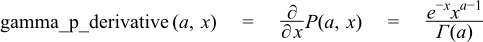#Boost C++ Libraries

...one of the most highly regarded and expertly designed C++ library projects in the world.

This is the documentation for an old version of boost. Click here for the latest Boost documentation.

#### Derivative of the Incomplete Gamma Function

##### Synopsis

```#include <boost/math/special_functions/gamma.hpp>
```

```namespace boost{ namespace math{

template <class T1, class T2>
calculated-result-type gamma_p_derivative(T1 a, T2 x);

template <class T1, class T2, class Policy>
calculated-result-type gamma_p_derivative(T1 a, T2 x, const Policy&);

}} // namespaces
```
##### Description

This function find some uses in statistical distributions: it implements the partial derivative with respect to x of the incomplete gamma function.The final Policy argument is optional and can be used to control the behaviour of the function: how it handles errors, what level of precision to use etc. Refer to the policy documentation for more details.

Note that the derivative of the function gamma_q can be obtained by negating the result of this function.

The return type of this function is computed using the result type calculation rules when T1 and T2 are different types, otherwise the return type is simply T1.

##### Accuracy

Almost identical to the incomplete gamma function gamma_p: refer to the documentation for that function for more information.

##### Implementation

This function just expose some of the internals of the incomplete gamma function gamma_p: refer to the documentation for that function for more information.